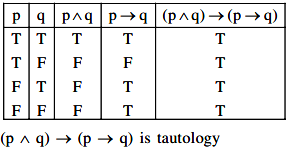# Which of the following Boolean expression is a tautology? (1) (p ∧ q) ∨ (p ∨ q)

Which of the following Boolean expression is a tautology?

(1) (p ∧ q) $$\vee$$ (p $$\vee$$ q)

(2) (p ∧ q) $$\vee$$ (p → q)

(3) (p ∧ q) ∧ (p → q)

(4) (p ∧ q) → (p → q)

verified

Correct Answer is 4) (p ∧ q) → (p → q)